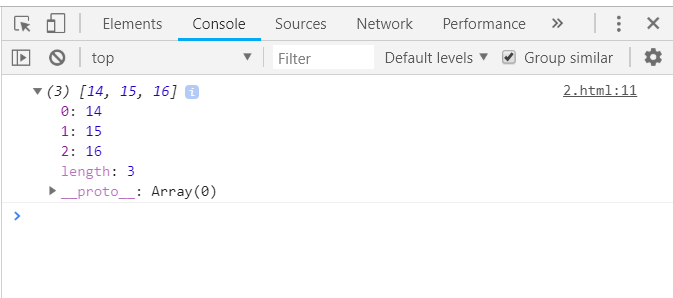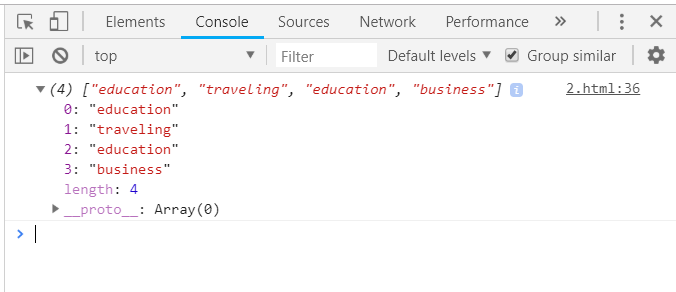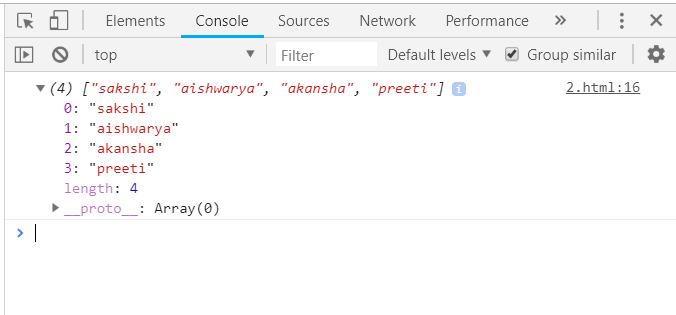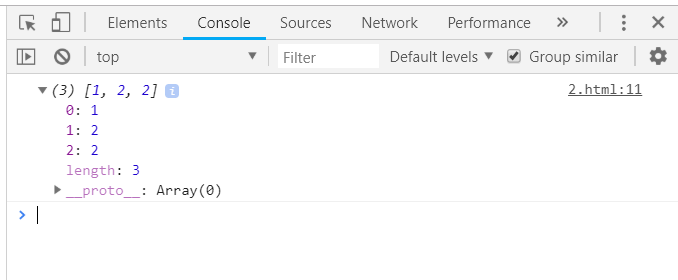Skip to content
Related Articles
Underscore.js | pluck() with Examples
• Last Updated : 21 Oct, 2020

The Underscore.js is a JavaScript library that provides a lot of useful functions that helps in the programming in a big way like the map, filter, invoke, etc even without using any built-in objects.

The _.pluck() function is used when we need to extract a list of a given property. Like we have to find out the name of all the students, then we can simply apply the _.pluck() function on the details of all the students. It will only extract the name from the details of all the stuf=dents and display it. The hence formed list will be an array of names only.

Syntax:

`_.pluck(list, propertyName) `

Parameters: It takes two arguments:

• List
• Property Name: It is the property on which we need to aggregate the content.

Return values:
The returned value is an array of that property’s detail which we need to extract. The array will contain the elements in the same order in which they were in the list.

• Extracting a number property from the _.pluck function():
The _.pluck() function takes the element from the list one by one and starts extracting the given property’s detail starting from the first element. Like here the operation is to find all the age in the list.So, the output will be a number array containing all the ages of the elements.
 `<``html``>``  ` `<``head``>``    ``<``script` `src` `= ``"https://cdnjs.cloudflare.com/ajax/libs/underscore.js/1.9.1/underscore-min.js"` `>``    ``````  ` `<``body``>``    ``<``script` `type``=``"text/javascript"``>``          ``var list = [{name: 'jack', age: 14}, {name: 'jill', age: 15},``                             ``{name: 'humpty', age: 16}];``          ``console.log(_.pluck(list, 'age'));``    ``````  ` ``

Output:• Extracting a string property from the _.pluck() function:
Firstly, define the array with all the elements having complete details. Then pass the property on the basis of which you need to separate the details. Like here ‘category’ property is used. So, al types of categories from the list will be displayed as a new array.
 ```<``html``>``  ` `<``head``>``    ``<``script` `src` `= ``"https://cdnjs.cloudflare.com/ajax/libs/underscore.js/1.9.1/underscore-min.js"` `>``    ``````  ` `<``body``>``    ``<``script` `type``=``"text/javascript"``>``         ``var goal  = [``        ``{``            ``"category" : "other",``            ``"title" : "harry University",``            ``"value" : 50000,``            ``"id":"1"``        ``},``        ``{``            ``"category" : "traveling",``            ``"title" : "tommy University",``            ``"value" : 50000,``            ``"id":"2"``        ``},``        ``{``            ``"category" : "education",``            ``"title" : "jerry University",``            ``"value" : 50000,``            ``"id":"3"``        ``},``        ``{    ``            ``"category" : "business",``            ``"title" : "Charlie University",``            ``"value" : 50000,``            ``"id":"4"``        ``}``    ``]``    ``console.log(_.pluck(goal, 'category'));``    ``````  ` ``

Output:• Extracting the ‘name’ property from the _.invoke() function: (most common use)
Follow the same steps like firstly, defining the complete array and then passing the array name with its property which needs to be extracted. The output array will contain all the names from the list.
 ```<``html``>``  ` `<``head``>``    ``<``script` `src` `= ``"https://cdnjs.cloudflare.com/ajax/libs/underscore.js/1.9.1/underscore-min.js"` `>``    ``````  ` `<``body``>``    ``<``script` `type``=``"text/javascript"``>``         ``var people = [``        ``{"name": "sakshi", "hasLong": "false"},``        ``{"name": "aishwarya", "hasLong": "true"},``        ``{"name": "akansha", "hasLong": "true"},``        ``{"name": "preeti", "hasLong": "true"}``    ``]``     ``console.log(_.pluck(people, 'name'););``    ``````  ` ``

Output:• Extracting property when that property repeats:
Create a array contain a property which has the same value for atleast two elements. Then, if we pass the array and that property to the _.pluck() function, it will display all the possible property’s detail despite of the fact that it is repeating or not.
 `<``html``>``  ` `<``head``>``    ``<``script` `src` `= ``   ``"https://cdnjs.cloudflare.com/ajax/libs/underscore.js/1.9.1/underscore-min.js"``>``````  ` `<``body``>``    ``<``script` `type``=``"text/javascript"``>``         ``var users = [{id: 1, name:"harry"}, {id: 2, name:"jerry"},``                 ``{id: 2, name:"jack"}];``    ``console.log(_.pluck(users, 'id'));``    ``````  ` ``

Output:NOTE: These commands will not work in Google console or in firefox as for these, additional files need to be added which they didn’t have added.
So, add the below links to your HTML file and then run them.

 ```<``script` `type``=``"text/javascript"` `src` `=``"https://cdnjs.cloudflare.com/ajax/libs/underscore.js/1.9.1/underscore-min.js"``>```

An example is shown below:

 `<``html``>``  ` `<``head``>``    ``<``script` `type``=``"text/javascript"` `src` `=``     ``"https://cdnjs.cloudflare.com/ajax/libs/underscore.js/1.9.1/underscore-min.js"``>``     ``````  ` `<``body``>``    ``<``script` `type``=``"text/javascript"``>``         ``var users = [{id: 1, name:"harry"}, {id: 2, name:"jerry"},``                                  ``{id: 2, name:"jack"}];``    ``console.log(_.pluck(users, 'id'));``    ``````  ` ``

My Personal Notes arrow_drop_up